# How to Calculate and Solve for Glide Shear | Fracture Mechanics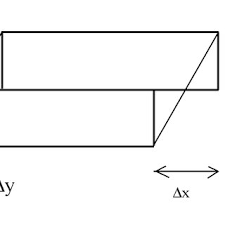The image above represents glide shear.

To compute for glide shear, two essential parameters are needed and these parameters are Stacking Fault Energy (γ) and Varying Grain Constant (M’).

The formula for calculating glide shear:

E = γ / M’

Where:

E = Glide Shear
γ = Stacking Fault Energy
M’ = Varying Grain Constant

Let’s solve an example;
Find the glide shear when the stacking fault energy is 18 and the varying grain constant is 2.

This implies that;

γ = Stacking Fault Energy = 18
M’ = Varying Grain Constant = 2

E = γ / M’
E = 18 / 2
E = 9

Therefore, the glide shear is 9 Pa.

Calculating the Stacking Fault Energy when the Glide Shear and the Varying Grain Constant is Given.

γ = E x M’

Where;

γ = Stacking Fault Energy
E = Glide Shear
M’ = Varying Grain Constant

Let’s solve an example;
Find the stacking fault energy when the glide shear is 14 and the varying grain constant is 8.

This implies that;

E = Glide Shear = 14
M’ = Varying Grain Constant = 8

γ = E x M’
γ = 14 x 8
γ = 112

Therefore, the stacking fault energy is 112.

Calculating the Varying Grain Constant when the Glide Shear and the Stacking Fault Energy is Given.

M’ = γ / E

Where;

M’ = Varying Grain Constant
E = Glide Shear
γ = Stacking Fault Energy

Let’s solve an example;
Find the varying grain constant when the glide shear is 10 and the stacking fault energy is 40.

This implies that;

E = Glide Shear = 10
γ = Stacking Fault Energy = 40

M’ = γ / E
M’ = 40 / 10
M’ = 4

Therefore, the varying grain constant is 4.

Nickzom Calculator – The Calculator Encyclopedia is capable of calculating the glide shear.

To get the answer and workings of the glide shear using the Nickzom Calculator – The Calculator Encyclopedia. First, you need to obtain the app.

You can get this app via any of these means:

To get access to the professional version via web, you need to register and subscribe for NGN 2,000 per annum to have utter access to all functionalities.
You can also try the demo version via https://www.nickzom.org/calculator

Apple (Paid) – https://itunes.apple.com/us/app/nickzom-calculator/id1331162702?mt=8
Once, you have obtained the calculator encyclopedia app, proceed to the Calculator Map, then click on Materials and Metallurgical under Engineering.Now, Click on Fracture Mechanics under Materials and MetallurgicalNow, Click on Glide Shear under Fracture Mechanics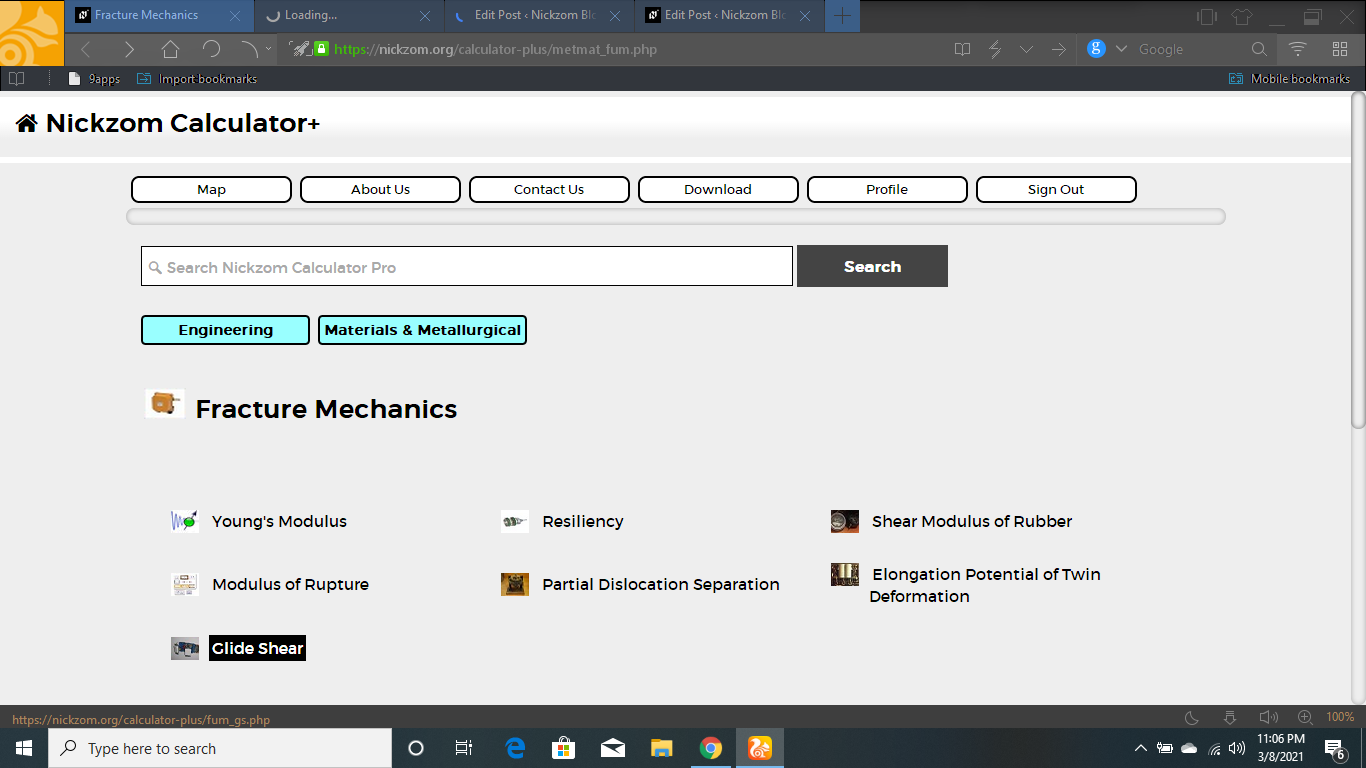The screenshot below displays the page or activity to enter your values, to get the answer for the glide shear according to the respective parameters which is the Stacking Fault Energy (γ) and Varying Grain Constant (M’).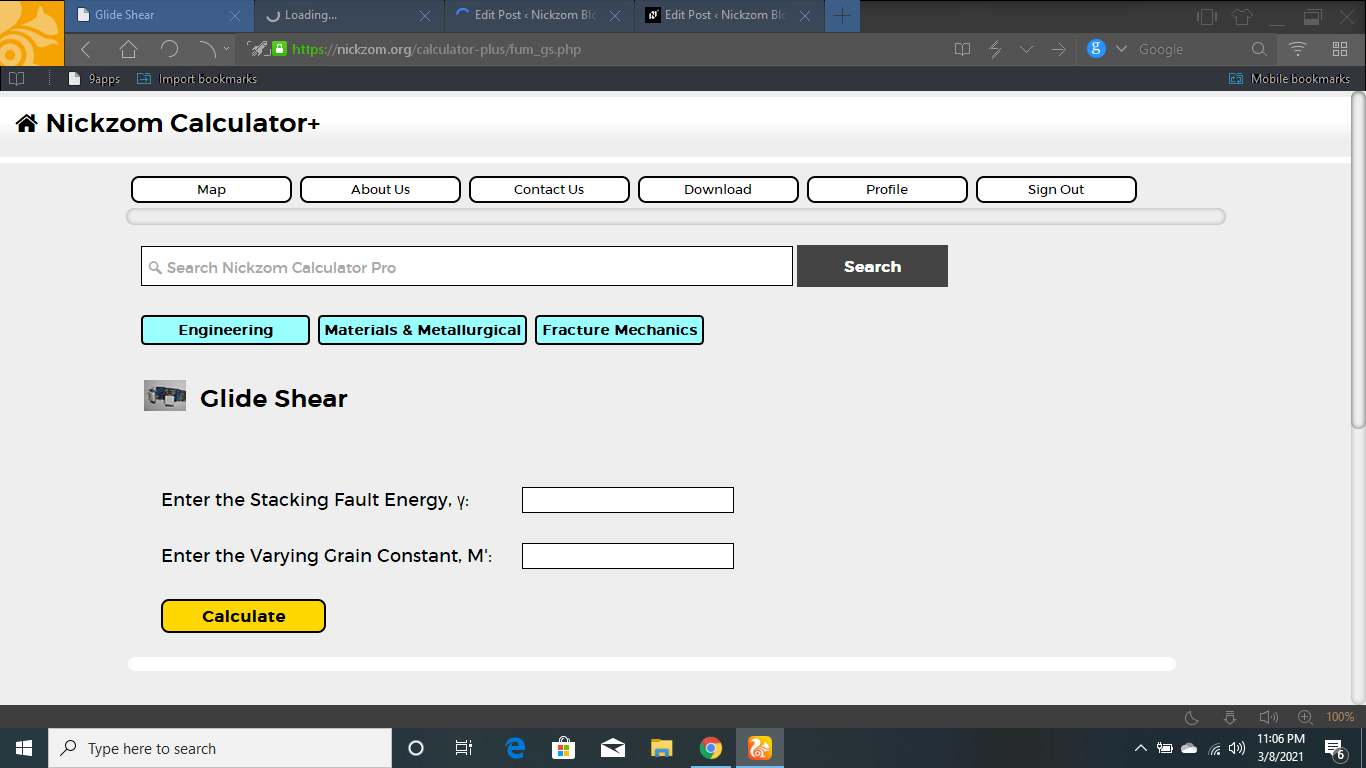Now, enter the value appropriately and accordingly for the parameter as required by the Stacking Fault Energy (γ) is 18 and Varying Grain Constant (M’) is 2.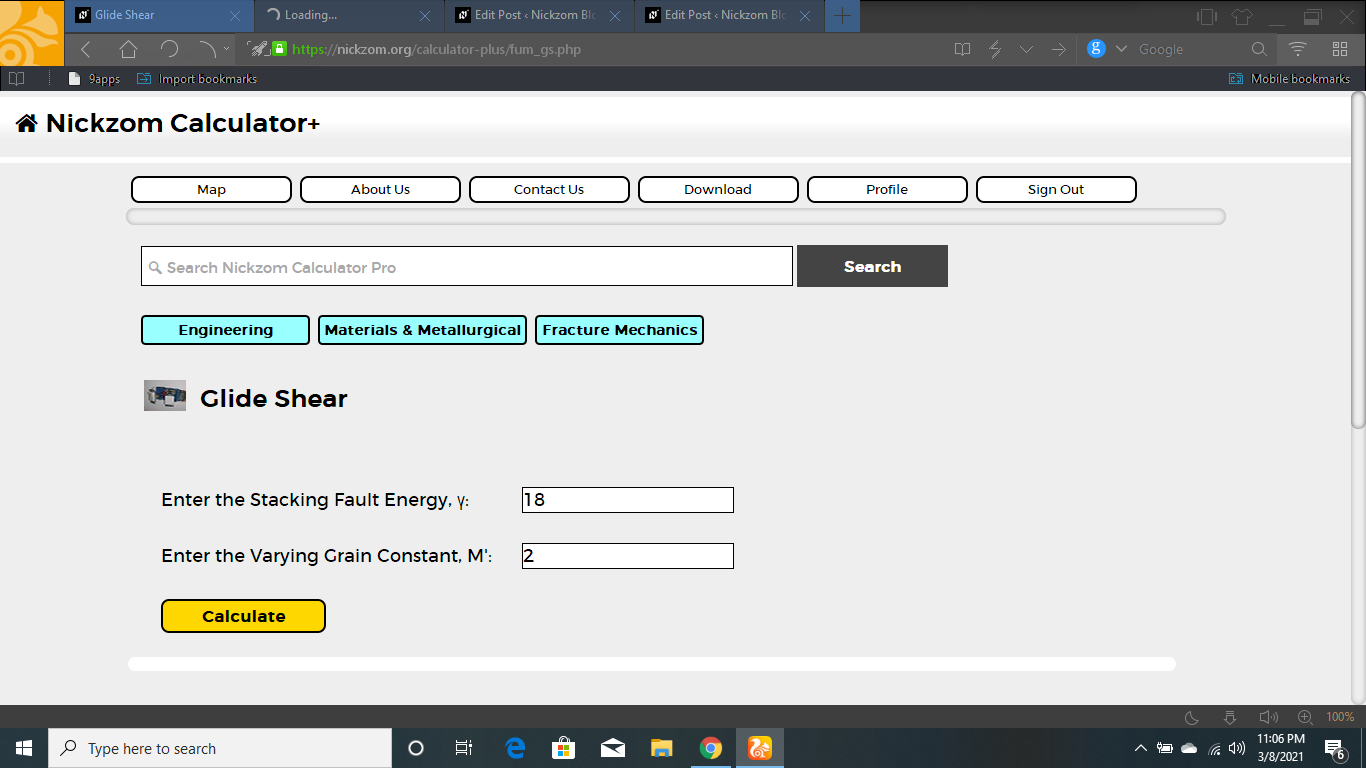Finally, Click on Calculate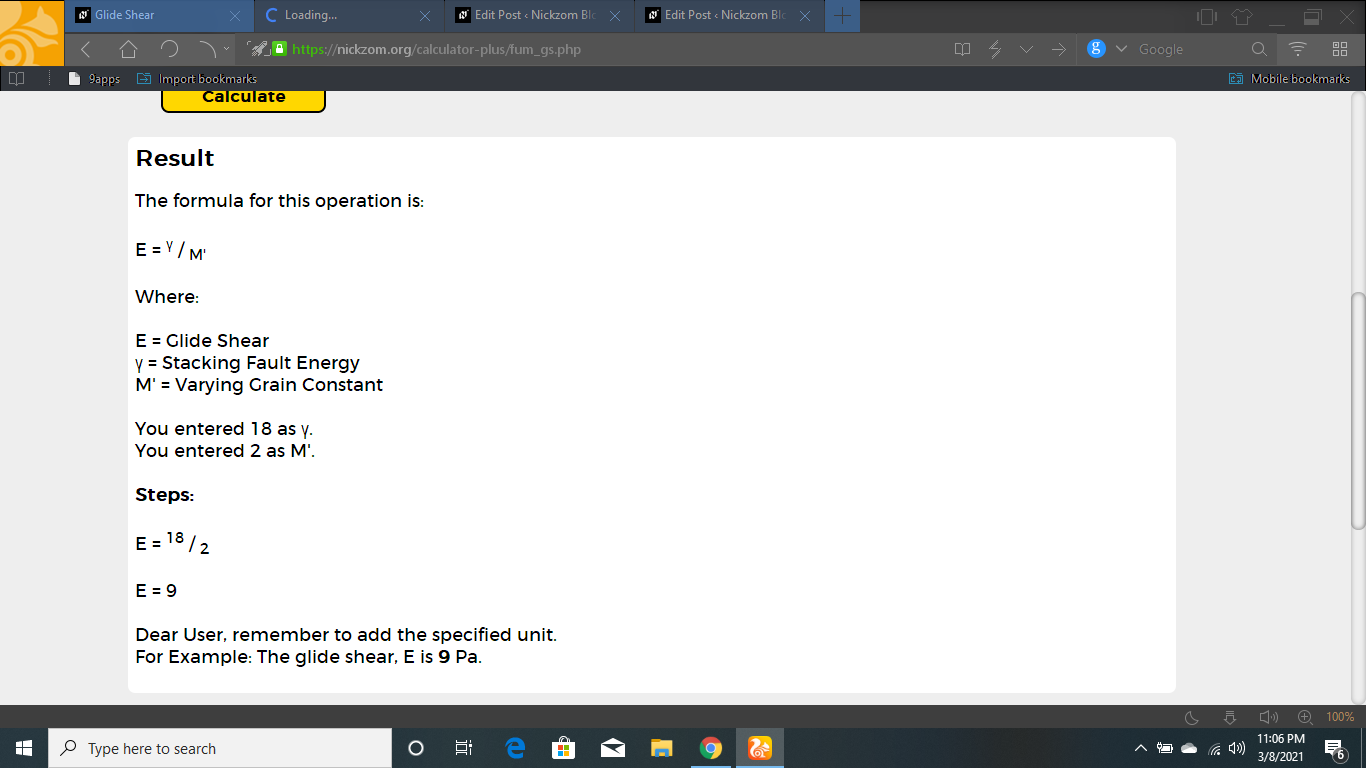As you can see from the screenshot above, Nickzom Calculator– The Calculator Encyclopedia solves for the glide shear and presents the formula, workings and steps too.